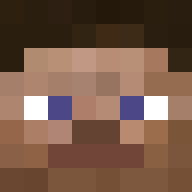Last Activity:
Apr 11, 2019
Joined:
Nov 26, 2017
Messages:
46
4

Followers2

Gender:
Male
Birthday:
Apr 21, 1996 (Age: 23)

RblsGxBoygone

Junior Member, Male, 23

RblsGxBoygone was last seen:
Apr 11, 2019
1. There are no messages on RblsGxBoygone's profile yet.

Gender:
Male
Birthday:
Apr 21, 1996 (Age: 23)

Content:

Signature

==+==+==+==+==+==+==+==+==+==+==+==+==+==+==+==+==

RblsGxBoygoneGaming

Quote: You miss 100% of the shots you never takes - Wayne Gretzky

==+==+==+==+==+==+==+==+==+==+==+==+==+==+==+==+==